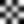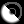# Convolution node

Left Toolbar >Filter Nodes >Convolution node

The Convolution node applies a convolution filter to the image, replacing each pixel with a value that is determined by adding the weighted values of all its neighbors together.

inputImg

outputImage with a edges all convolution filter of 3 x 3 pixels.

## Procedure

### To add a convolution filter to an image:

1. On the left toolbar choose Filter Nodes > Convolution.>2. Drag the Convolution icon into the Node Graph.3. Connect the output of an image node into the image input in the Convolution node.

4. Adjust the attributes of the Convolution node to modify the output image.

## Inputs

image
The Image to be processed by the convolution filtered.

## Outputs

image
The processed image.

## Attributes PanelFilter
The filter to be applied.
Edges Up. Finds the edges from the top.
Edges Down: Finds the edges from the bottom.
Edges Right: Finds the edges from the right.
Edges Left: Finds the edges from the left.
Edges All: Finds all edges.
Sharpen: Sharpens the image.
Motion Blur 45: Applies a motion blur from bottom-left to top-right.
Motion Blur 135: Applies a motion blur from top-left to bottom-right.

Size
Size of the kernel matrix.
3: 3x3
5: 5x5

Boundary
The boundary conditions of the emboss filter.
Seamless: Pixels are repeated from the other side of the image, generating an output image that can be tiled.
Mirror: Pixels are mirrored from the same side of the image.
Border: Border pixels are repeated.

Keep Opacity
If checked, doesn't apply the filter to the opacity channel.

Filter Nodes

Main Page